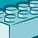# New to Qlik Sense

If you’re new to Qlik Sense, start with this Discussion Board and get up-to-speed quickly.

Announcements
QlikWorld Online 2021, May 10-12: Our Free, Virtual, Global Event REGISTER TODAY
cancel
Showing results for
Search instead for
Did you mean:Contributor III

## Current Year (Sum)

I use this formula to calculate sum for the current year. .

if(GetSelectedCount ([Month])> 0 or GetSelectedCount(Year)> 0, Sum([g.SellingAmount]), Sum ({<Date = P({<Year={\$(=max(Year(today())))}>}Date)>}[g.SellingAmount]))

The field g.sellingAmount has different values and how can I change this formula to access only one of them. I want to calculate the sum for the current year only for one of them..

1 Solution

Accepted SolutionsCreator III

You can use Name1 inside your set analysis

if(GetSelectedCount ([Month])> 0 or GetSelectedCount(Year)> 0, Sum([g.SellingAmount]), Sum ({<Name1={*},Date = P({<Year={\$(=max(Year(today())))}>}Date)>}[g.SellingAmount]))

this will caluclate for name 1 column's too.

Many Thanks

Karthik

4 RepliesMaster II

Hi,

try below method,

u can simply try to use set analysis expression like below

Sum ({\$<Year ={"\$(=Max(Year))"} >} [g.SellingAmount])

or

u can try to add current year YTD flag in your calendar script

LET vMinDate = num(Peek('MinDate'));

LET vMaxDate = num(Peek('MaxDate'));

LET vToday = vMaxDate;

InYearToDate(CalDate,\$(vToday),0) * -1 as CurrYTDFlag,

InYearToDate(CalDate,\$(vToday),-1) * -1 as LastYTDFlag,

then u can use it

Sum ({\$<CurrYTDFlag={1} >} [g.SellingAmount])

Thanks,DevaContributor III
Author

Thank you for your help.but I have a different question.

For example, in this field we have 'Name1', 'Name2', 'Name3','Name4',columns and I want to calculate only for 'Name1''s sum for the current year.

In this formula, all values are calculated. and I want to change this in such way to show only one of them.

if(GetSelectedCount ([Month])> 0 or GetSelectedCount(Year)> 0, Sum([g.SellingAmount]), Sum ({<Date = P({<Year={\$(=max(Year(today())))}>}Date)>}[g.SellingAmount]))Creator III

You can use Name1 inside your set analysis

if(GetSelectedCount ([Month])> 0 or GetSelectedCount(Year)> 0, Sum([g.SellingAmount]), Sum ({<Name1={*},Date = P({<Year={\$(=max(Year(today())))}>}Date)>}[g.SellingAmount]))

this will caluclate for name 1 column's too.

Many Thanks

KarthikContributor III
Author

Thank you very muchIt worksTags